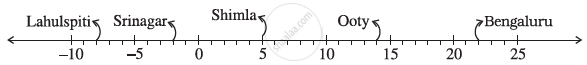Share

# Following Number Line Shows the Temperature in Degree Celsius (°C) at Different Places on a Particular Day. Can We Say Temperature of Srinagar and Shimla Taken Together is Less than the Temperature at Shimla? is It Also Less than the Temperature at Srinagar? - CBSE Class 7 - Mathematics

ConceptIntroduction of Integer

#### Question

Following number line shows the temperature in degree celsius (°C) at different places on a particular day.Can we say temperature of Srinagar and Shimla taken together is less than the temperature at Shimla? Is it also less than the temperature at Srinagar?

#### Solution

Temperature at Srinagar = −2°C

Temperature at Shimla = 5°C

Temperature of Srinagar and Shimla taken together = −2°C + 5°C

= 3°C

3°C < 5°C

3°C < Temperature of Shimla

Yes, the temperature of Srinagar and Shimla taken together is less than the temperature of Shimla.

However, 3°C > −2°C

Hence, the temperature of Srinagar and Shimla taken together is not less than the temperature of Srinagar.

Is there an error in this question or solution?

#### APPEARS IN

NCERT Solution for Mathematics for Class 7 (2018 to Current)
Chapter 1: Integers
Ex. 1.10 | Q: 1.4 | Page no. 4
Solution Following Number Line Shows the Temperature in Degree Celsius (°C) at Different Places on a Particular Day. Can We Say Temperature of Srinagar and Shimla Taken Together is Less than the Temperature at Shimla? is It Also Less than the Temperature at Srinagar? Concept: Introduction of Integer.
S